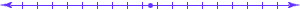## Challenge Problems in Automata and Formal Languages

### Revised October 30, 2005.

The purpose of these problems is to provide a challenge to the top students, meaning those who master the regular material of the course and would like to learn more.
Do not try to work these problem to improve a poor grade ... if you are doing badly in the course, concentrate on the regular material.
• Fix a constant n. Let L1 be the the language over the alphabet {a,b} consisting of all strings of the form uav where u and v are strings and |u| = n. Let L2 be the the language over the alphabet {a,b} consisting of all strings of the form uav where u and v are strings and |v| = n.
1. Give an NFA with n+2 states that accepts L1.
2. Prove that no NFA with fewer than n+2 states can accept L1, or find one with fewer states.
3. Give an NFA with n+2 states that accepts L2.
4. Prove that no NFA with fewer than n+2 states can accept L2, or find one with fewer states.
5. Give a DFA with n+2 states that accepts L1.
6. Prove that the minimal DFA that accepts L2 has exactly 2n+1 states.
• Some people say you "can't get" pi, that is, the well-known ratio of the circumference of a circle to its diameter. Are they right? What does that even mean? Work these problems.
• Prove that, if P = NP, RSA coding is insecure. (You must, of course, first define "insecure.")
What is a public-key encryption system? Give a sensible, mathematically rigorous, definition.
Is it true that, if P = NP, public-key encryption is impossible?
• Let Lhalt = {<M>w | M accepts w}.
We know that Lhalt is undecidable, but if M is simple and w is short, we would intuitively think that, if M eventually halts with input w, it shouldn't take too many steps. However, this intuition is wrong.
Prove the following theorem:
If f is any recursive function, there is a number n and a string <M>w in Lhalt of length no more than n, such that M does not halt with input w in the first f(n) steps.Turing Machine Emulation